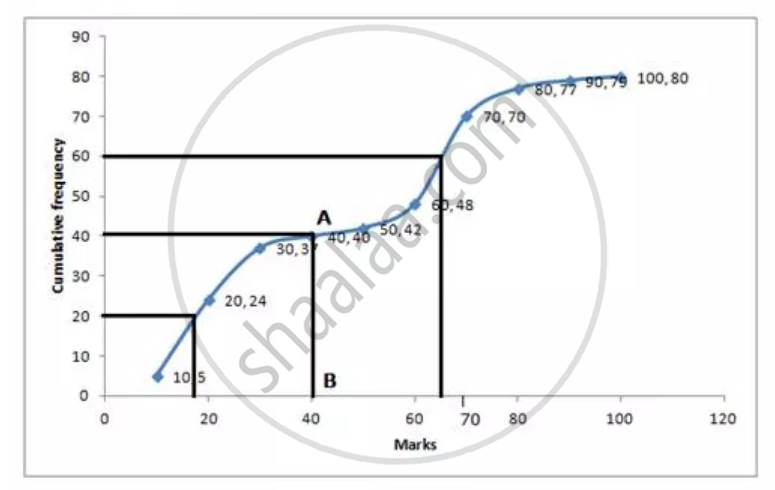Share
Notifications

View all notifications
Books Shortlist
Your shortlist is empty

# Solution for From the Following Cumulative Frequency Table , Find : Median Lower Quartile Upper Quaetile - ICSE Class 10 - Mathematics

Login
Create free account

Forgot password?
ConceptMeasures of Central Tendency - Mean, Median, Mode for Raw and Arrayed Data

#### Question

From the following cumulative frequency table , find :

Median

Lower quartile

Upper quaetile

 Marks (less than ) 10 20 30 40 50 60 70 80 90 100 Cumulative frequency 5 24 37 40 42 48 70 77 79 80

#### Solution

 Marks (less than) Cumulative frequency 10 5 20 24 30 37 40 40 50 42 60 48 70 70 80 77 90 79 100 80

Number of term= 80

\ Median= 40^(th) termMedian= Through 40^(th) term mark draw a line parallel to the x-axis which meets the curve at A. from A, Draw a perpendiculer to axis which meets it at B.

Value of B is the median -40

Lower quartile (Q_1)=20^(th) term=18

Upeer Quartile (Q_3)=60^th term=66

Is there an error in this question or solution?

#### APPEARS IN

Selina Solution for Selina ICSE Concise Mathematics for Class 10 (2017 to Current)
Chapter 24: Measure of Central Tendency(Mean, Median, Quartiles and Mode)
Q: 9
Solution From the Following Cumulative Frequency Table , Find : Median Lower Quartile Upper Quaetile Concept: Measures of Central Tendency - Mean, Median, Mode for Raw and Arrayed Data.
S